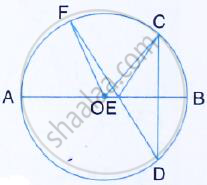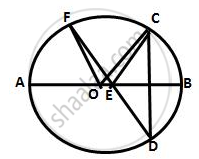Share

Books Shortlist

# In the Figure Given Below, Ab is Diameter of the Circle Whose Centre is O. Given That: ∠Ecd = ∠Edc = 32°. Show that ∠Cof = ∠Cef. - ICSE Class 10 - Mathematics

ConceptArc and Chord Properties - the Angle that an Arc of a Circle Subtends at the Center is Double that Which It Subtends at Any Point on the Remaining Part of the Circle

#### Question

In the figure given below, AB is diameter of the circle whose centre is O. given that: ∠ECD =
∠EDC = 32°. Show that ∠COF = ∠CEF.#### SolutionHere, ∠COF = 2 ∠CDF = 2 × 32° = 64°  ……… (i)
(Angle at the centre is double the angle at the circumference subtended by the same chord)
In ΔECD,
∠CEF = ∠ECD +∠EDC = 32° +32°  = 64°  ………….(ii)
(Exterior angle of a Δ is equal to the sum of pair of interior opposite angles)
From (i) and (ii), we get
∠COF =∠CEF

Is there an error in this question or solution?

#### Video TutorialsVIEW ALL 

Solution In the Figure Given Below, Ab is Diameter of the Circle Whose Centre is O. Given That: ∠Ecd = ∠Edc = 32°. Show that ∠Cof = ∠Cef. Concept: Arc and Chord Properties - the Angle that an Arc of a Circle Subtends at the Center is Double that Which It Subtends at Any Point on the Remaining Part of the Circle.
S